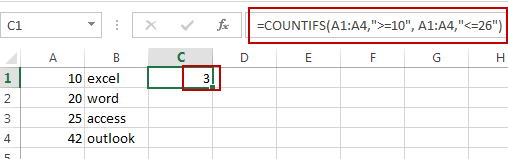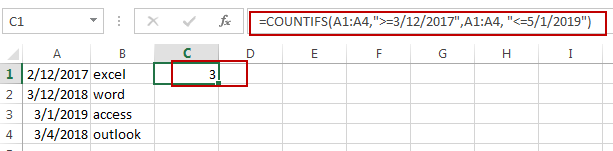# How to Count Cells between Two Numbers or Dates in Excel

This post will guide you how to count the number of cells between two numbers or dates in Excel. How do I count numbers or dates based on a conditional in Excel. How to count cells between two numbers with a formula in Excel.

## Count Cells between Two Numbers

Assuming that you have a list of data in range A1:B4, which contain number values in column A, and you want to count the number of cells between two given numbers (10 and 20) in Excel. How to achieve it. You can use a formula based on the COUNTIFS function to achieve the result. Like this:

`=COUNTIFS(A1:A4,">=10", A1:A4,"<=26")`

Type this formula into a blank cell and then press Enter key in your keyboard. The cell number will be counted.## Count Cells between Two Dates

If you want to count the number of cells between two dates (3/12/2017 and 5/1/2019) in Excel, you can also use a formula based on the COUNTIFS function to achieve the result. Like this:

`=COUNTIFS(A1:A4,">=3/12/2017",A1:A4, "<=5/1/2019")`

Type this formula into a blank cell and then press Enter key in your keyboard. The cell number between two given dates will be counted.### Related Functions

• Excel COUNTIFS function
The Excel COUNTIFS function returns the count of cells in a range that meet one or more criteria. The syntax of the COUNTIFS function is as below:= COUNTIFS(criteria_range1, criteria1, [criteria_range2, criteria2]…)…